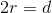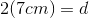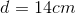Basic Geometry : How to find the length of the diameter

Example Questions

Example Question #51 : How To Find The Length Of The Diameter

Find the diameter of a circle whose radius is 5.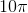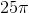Explanation:

The diameter of a circle is the width from side to side going through the center on the circle. In other words, the diameter is twice the radius.

To solve, simply use the formula for the diameter of a circle and substitute in the known radius in question.

Thus,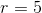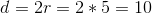Example Question #52 : How To Find The Length Of The Diameter

Find the length of the diameter of a circle given a radius of 7.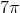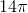Explanation:

The diameter of a circle is the width from side to side going through the center on the circle. In other words, the diameter is twice the radius.

To solve, simply use the formula for the diameter of a circle and substitute in the known radius in question.

Thus,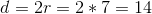Example Question #53 : How To Find The Length Of The Diameter

Penny recently moved into a Native American Teepee with a floor plan that is a perfect circle and encompasses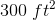. What would be the diameter of the circular floor plan for Penny's Teepee? Round your value to the nearest tenth.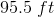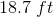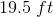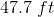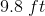Explanation:

Since we already know the area of the circle, we can look at the equation for circular area to find the diameter: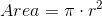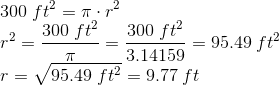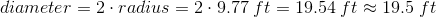Example Question #54 : How To Find The Length Of The Diameter

Find the length of the diameter of a circle with radius 100.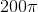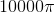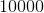Explanation:

To solve, simply use the formula for the diameter. Thus,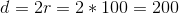Don't be fooled by large numbers, simply use the formula how you memorized it. Remember, diameter is just twice the radius.

Example Question #55 : How To Find The Length Of The Diameter

A circle has a circumference of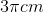. What is the length of the circle's diameter?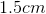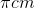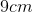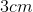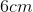Explanation:

The formula for the circumference of a circle is: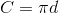Since the circumference is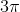: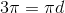We then solve for: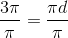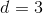Therefore, the circle's diameter is.

Example Question #56 : How To Find The Length Of The Diameter

A circle has an area of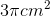. What is the diameter of the circle?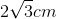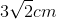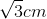Explanation:

The formula for the area of a circle is:Since we know the area is, we plug that in to solve for the radius: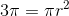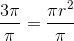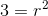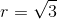Now that we know the radius, we simply double it to find the diameter: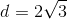So the diameter of the circle is.

Example Question #57 : How To Find The Length Of The Diameter

From the center of a circle playing field to the edge is 25 yards. This is called the radius. What is the length of the diameter of the field?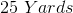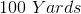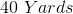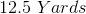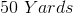Explanation:

To find the diameter of a circle, it is from one side of the circle through the center of the circle to the other side. Or if the radius is from the center of a circle to the edge, the diameter must be twice the radius.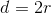Since the information given is the radius of the circle we know that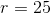.

Substituting the radius into the above equation we can solve for the diameter.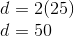Therfore the diameter of this circle is double 25 yards which would be 50 yards.

Example Question #58 : How To Find The Length Of The Diameter

The top of a can has a radius of 5cms. What is the length of the diameter of the can?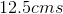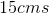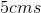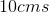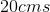Explanation:

To find the diameter of a circle, it is from one side of the circle through the center of the circle to the other side. Or if the radius is from the center of a circle to the edge, the diameter must be twice the radius.Since the information given is the radius of the circle we know that.

Substituting the radius into the above equation we can solve for the diameter.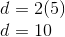Therfore the diameter of this circle is double 5cms which would be 10cms.

Example Question #59 : How To Find The Length Of The Diameter

A circular pool has a circumference of 27 meters. What the diameter of the pool?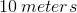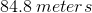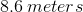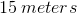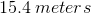Explanation:

We find the circumference of circles with the relationship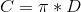, where D is diameter.

Since we are given the circumference of the pool, solve for D by dividing both sides by.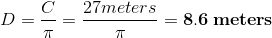Example Question #60 : How To Find The Length Of The Diameter

If the radius of a circle is 7cm, what is the diameter?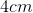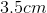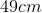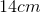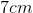Explanation:

The radius is half of the diameter.